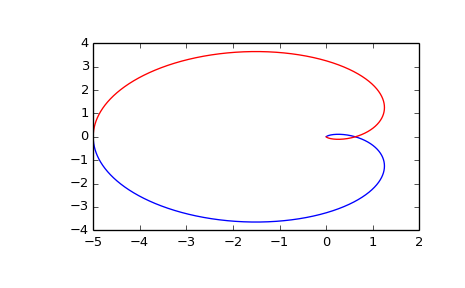# scipy.signal.freqresp¶

scipy.signal.freqresp(system, w=None, n=10000)[source]

Calculate the frequency response of a continuous-time system.

Parameters: system : an instance of the LTI class or a tuple describing the system. The following gives the number of elements in the tuple and the interpretation: 2 (num, den) 3 (zeros, poles, gain) 4 (A, B, C, D) w : array_like, optional Array of frequencies (in rad/s). Magnitude and phase data is calculated for every value in this array. If not given a reasonable set will be calculated. n : int, optional Number of frequency points to compute if w is not given. The n frequencies are logarithmically spaced in an interval chosen to include the influence of the poles and zeros of the system. w : 1D ndarray Frequency array [rad/s] H : 1D ndarray Array of complex magnitude values

Examples

# Generating the Nyquist plot of a transfer function

>>> from scipy import signal
>>> import matplotlib.pyplot as plt

>>> s1 = signal.lti([], [1, 1, 1], )
# transfer function: H(s) = 5 / (s-1)^3

>>> w, H = signal.freqresp(s1)

>>> plt.figure()
>>> plt.plot(H.real, H.imag, "b")
>>> plt.plot(H.real, -H.imag, "r")
>>> plt.show()#### Previous topic

scipy.signal.bessel

scipy.signal.lti